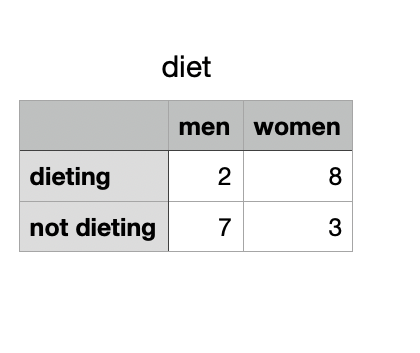GFG App
Open AppBrowser
Continue

# How to Perform Fisher’s Exact Test in Python

Fisher’s exact test is a statistical test that determines if two category variables have non-random connections or we can say it’s used to check whether two category variables have a significant relationship. In this article let’s learn how to perform Fisher’s exact test. In python fisher exact test can be performed using the fisher_exact() function from the SciPy library.

Syntax: scipy.stats.fisher_exact(table, alternative=’two-sided’)

Parameters:

table : array like 2X2 contigency table. negative integers aren’t allowed.

alternative:  it’s an optional value which represents the alternative hypothesis. values can be {‘two-sided’, ‘less’, ‘greater’}, by default it is two sided

• less : one sided
• greater: one sided

Returns : oddratio and p_value.

Types of hypothesis

• null hypothesis:  no associations between the rows and columns, or the classifications are independent.
• alternative hypothesis:  there are associations between the rows and columns, or the classifications are dependent.

The 2X2 contingency table we use for the example is :

2 men and 8 women follow a diet. 7 men and 3 women don’t.## Python3

 `# importing packages ` `import` `scipy.stats as stats ` ` `  `# creating data ` `data ``=` `[[``2``, ``8``], [``7``, ``3``]] ` ` `  `# performing fishers exact test on the data ` `odd_ratio, p_value ``=` `stats.fisher_exact(data) ` `print``(``'odd ratio is : '` `+` `str``(odd_ratio)) ` `print``(``'p_value is : '` `+` `str``(p_value)) `

Output:

```odd ratio is : 0.10714285714285714
p_value is : 0.06977851869492728```

if we take the level of significance to be 0.05, we fail to reject the null hypothesis as the p_value is above 0.05. the alternative hypothesis is rejected.

My Personal Notes arrow_drop_up